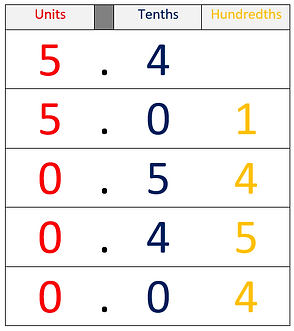top of page

## Topic 8: Place value (Decimals)Integers are whole numbers, but most numbers are non-integers. The numbers between integers are called decimals. Here are just some some of the decimals that lie between the integers 1 and 2.

1.1 is "one point one".  1.2 is "one point two", and so on.

The dot in a decimal number is called a decimal point.

The decimals shown above all have one decimal place. This means that there is one digit after the decimal point.

But decimals can have any number of decimal places. The decimals shown below have 2 decimal places. and are found between the decimals 1.1 and 1.2.

Using many decimal places allows us to make very precise measurements.  The decimals shown below have 3 decimal places, and are found between the decimals 1.15 and 1.16.

The 'columns' of a decimal number show place value, in the same way that they do for integers.

The column to the left of the decimal point is the units column.  The column to the right of the decimal point is the tenths column, and next column to right is the hundredths column.

In the number shown above, the digit 6 has a value of 6 units.

The digit 4 has a value of 4 tenths (4/10 or 0.4).

The digit 1 has a value of 1 hundredth (1/100 or 0.01).

You may be given a list of decimals like this one, and asked to put them in size order:

5.4           0.45           0.54           0.04           5.01

If you write the numbers in columns, it may be easier to see the order they should be in. Here is the correct order:

The number that may be confusing is 5.4, because it only has one decimal place.

The hundredths column in 5.4 is empty, so it could be marked with a zero (5.40).

This gives all of the numbers two decimal places, and should help with ordering the list:

5.40     >     5.01     >     0.54     >    0.45     >     0.04bottom of page